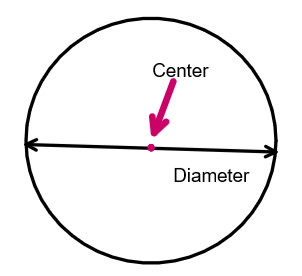Courses
Courses for Kids
Free study material
Offline Centres
MoreLast updated date: 02nd Dec 2023
Total views: 280.8k
Views today: 7.80k

# What is the circumference of a circle whose diameter is $12$ yards?Verified
280.8k+ views
Hint: The diameter of the circle is the chord that passes through the center of the circle or in other words the longest chord in the circle is known as the diameter of the circle.
The circumference of the circle is defined as the total length of the circle and it is equal to $2\pi r$, where ‘r’ is the radius of the circle and double of the radius ($2r$) is equal to the diameter of the circle.
Formula used:
circumference of the circle $= 2\pi r$
Given: diameter of the circle = $12$ yards
To find: circumference of the circle

Complete step-by-step solution:
Step 1: As we know that the circumference of the circle is given by,
Circumference of the circle $= 2\pi r$, where ‘r’ is the radius of the circle
Now, it is given that the diameter of the circle is $12$ yards and as we know that the diameter of the circle is equal to twice the radius of the circle. Therefore, we have
$\text{Diameter} = 2 \times \text{radius}$
Radius = $\dfrac{\text{diameter}}{2}$
Step 2: Substituting the values in circumference formula, we get
Circumference of the circle$= 2\pi r$
$\text{Circumference} = 2 \times \pi \times \text{radius}$
$\text{Circumference} = 2 \times \pi \times \dfrac{\text{diameter}}{2}$
$\text{Circumference} = 2 \times \pi \times \dfrac{{12}}{2}$
Step 3: After multiplication we get
$\text{Circumference} = 2 \times \pi \times \dfrac{{12}}{2}$
$\text{Circumference} = 2 \times 3.14 \times 6$
$\text{Circumference} = 37.68$ yards Or $\text{Circumference} = (37.68 \times 91.44)cm = 3445.46cm$
Hence, circumference of the circle whose diameter is $12$ yards is equal to $37.68$ yards or $3445.46$ cm

Note: All chords that pass through the center of the circle are known as the diameter of the circle and half of the length of the diameter is known as the radius of the circle. Here we know the direct formula for circumference for a circle in terms of radius as $2\pi r$ where r is radius but we are given the diameter so we write the formula of circle in terms of diameter. There is no other way to find the circumference.